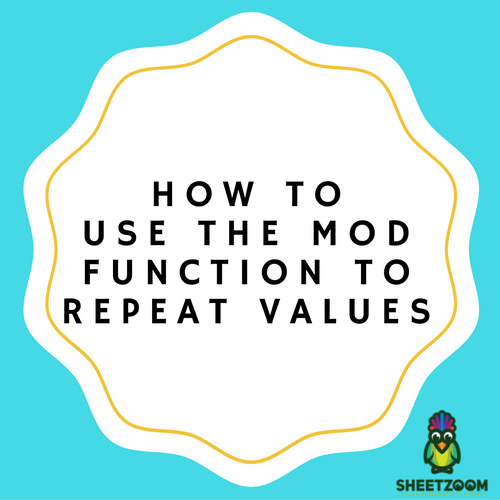# How To Use The MOD Function To Repeat ValuesHave you ever used the MOD function in Excel? Well, the function actually performs modulo operation. Input is basically two numbers, a divisor and number. The division is performed and reminder is returned. You might be thinking why you need to do this. Well, there are different ways in which a module is used.

Let’s start with a simple example. Suppose you maintain a budget properly and you would like to input some expenditure that occurs after every three months keeping the value zero in between the months. Now where to begin? What formula is there? Well, the MOD function is there to help you in this regard. You must have seen the remainder mentioned above, the actual trick here is not the reminder, it is actually that you don’t get a reminder or MOD returns zero. Nothing is there as a reminder so we can say that it goes evenly.

Our aim is to do something every 3rd month. Now, if the number is run through mod with 3 divisor and result we get is zero, we can simply add expenditure.

First of all, add the month number above the table. 1for January , 2 for February and so on. Now put simple formula.

MOD(cellnum, 3)

Now every 3rd month will get a value of 0. Using the IF statement, we can add values where the number is zero.

IF (MOD(Cellnum, 3 )= 0,60,0 )

Now, you will have 60 added at every third place and zero elsewhere. You have got what you wanted. It is the time to remove the numbers added above by you. For that you need to subtract 1 from each number. Now the numbers will appear as 2,3,4 and so on. Now problem, you can update the formula and get rid of this.

IF(MOD(COLUMN()-1,3)=0,\$B\$4,0)

And later you can delete the numbers.